# How to use INDIRECT function in Excel - formula examples

This Excel INDIRECT tutorial explains the function's syntax, basic uses and provides a number of formula examples that demonstrate how to use INDIRECT in Excel.

A great lot of functions exist in Microsoft Excel, some being easy-to-understand, other requiring a long learning curve, and the former being used more often than the latter. And yet, Excel INDIRECT is one of the kind. This Excel function does not perform any calculations, nor does it evaluate any conditions or logical tests.

Well then, what is the INDIRECT function in Excel and what do I use it for? This is a very good question and hopefully you will get a comprehensive answer in a few minutes when you've finished reading this tutorial.

## Excel INDIRECT function - syntax and basic uses

As its name suggests, Excel INDIRECT is used to indirectly reference cells, ranges, other sheets or workbooks. In other words, the INDIRECT function lets you create a dynamic cell or range reference instead of hard-coding them. As a result, you can change a reference within a formula without changing the formula itself. Moreover, these indirect references won't change when some new rows or columns are inserted in the worksheet or when you delete any existing ones.

All this may be easier to understand from an example. However, to be able to write a formula, even the simplest one, you need to know the function's arguments, right? So, let's have a quick look at Excel INDIRECT syntax first.

#### INDIRECT function syntax

The INDIRECT function in Excel returns a cell reference from a text string. It has two arguments, the first is required and the second is optional:

INDIRECT(ref_text, [a1])

ref_text - is a cell reference, or a reference to a cell in the form of a text string, or a named range.

a1 - is a logical value that specifies what type of reference is contained in the ref_text argument:

• If TRUE or omitted, ref_text is interpreted as an A1-style cell reference.
• If FALSE, ref_text is treated as a R1C1 reference.

While the R1C1 reference type might be useful in certain situations, you'll probably want to use the familiar A1 references most of the time. Anyway, nearly all INDIRECT formulas in this tutorial will use A1 references, so we will be omitting the second argument.

#### Basic use of the INDIRECT function

To get into the function's insight, let's write a simple formula that demonstrates how you use INDIRECT in Excel.

Suppose, you have number 3 in cell A1, and text A1 in cell C1. Now, put the formula `=INDIRECT(C1)` in any other cell and see what happens:

• The INDIRECT function refers to the value in cell C1, which is A1.
• The function is routed to cell A1 where it picks the value to return, which is number 3.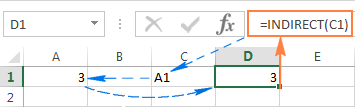So, what the INDIRECT function actually does in this example is converting a text string into a cell reference.

If you think this still has very little practical sense, please bear with me and I will show you some more formulas that reveal the real power of the Excel INDIRECT function.

## How to use INDIRECT in Excel - formula examples

As demonstrated in the above example, you can use the Excel INDIRECT function to put the address of one cell into another as a usual text string, and get the value of the 1st cell by referencing the 2nd. However, that trivial example is no more than a hint at the INDIRECT capabilities.

When working with real data, the INDIRECT function can turn any text string into a reference including very complex strings that you build using the values of other cells and results returned by other Excel formulas. But let's not put the cart before the horse, and run through several Excel Indirect formulas, one at a time.

### Creating indirect references from cell values

As you remember, the Excel INDIRECT function allows for A1 and R1C1 reference styles. Usually, you cannot use both styles in a single sheet at a time, you can only switch between the two reference types via File > Options > Formulas > R1C1 check box. This is the reason why Excel users rarely consider using R1C1 as an alternative referencing approach.

In an INDIRECT formula, you can use either reference type on the same sheet if you want to. Before we move any further, you may want to know the difference between A1 and R1C1 reference styles.

A1 style is the usual reference type in Excel that refers to a column followed by a row number. For example, B2 refers to the cell at the intersection of column B and row 2.

R1C1 style is the opposite reference type - rows followed by columns, which does take some time getting used to : ) For example, R4C1 refers to cell A4 which is in row 4, column 1 in a sheet. If no number comes after the letter, then you are referring to the same row or column.

And now, let's see how the INDIRECT function handles A1 and R1C1 references: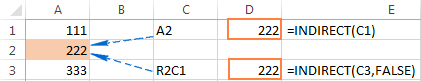As you see in the screenshot above, three different Indirect formulas return the same result. Have you already figured out why? I bet you have : )

• Formula in cell D1: `=INDIRECT(C1)`

This is the easiest one. The formula refers to cell C1, fetches its value - text string A2, converts it into a cell reference, heads over to cell A2 and returns its value, which is 222.

• Formula in cell D3: `=INDIRECT(C3,FALSE)`

FALSE in the 2nd argument indicates that the referred value (C3) should be treated like a R1C1 cell reference, i.e. a row number followed by a column number. Therefore, our INDIRECT formula interprets the value in cell C3 (R2C1) as a reference to the cell at the conjunction of row 2 and column 1, which is cell A2.

### Creating indirect references from cell values and text

Similarly to how we created references from cell values, you can combine a text string and a cell reference within your INDIRECT formula, tied together with the concatenation operator (&).

In the following example, the formula: =INDIRECT("B"&C2) returns a value from cell B2 based on the following logical chain:

The INDIRECT function concatenates the elements in the ref_text argument - text B and the value in cell C2 -> the value in cell C2 is number 2, which makes a reference to cell B2 -> the formula goes to cell B2 and returns its value, which is number 10.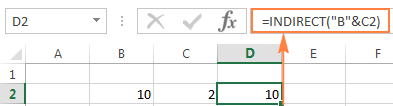### Using the INDIRECT function with named ranges

Apart from making references from cell and text values, you can get the Excel INDIRECT function to refer to named ranges.

Suppose, you have the following named ranges in your sheet:

• Apples - B2:B6
• Bananas - C2:C6
• Lemons - D2:D6

To create an Excel dynamic reference to any of the above named ranges, just enter its name in some cell, say G1, and refer to that cell from an Indirect formula `=INDIRECT(G1)`.

And now, you can take a step further and imbed this INDIRECT formula into other Excel functions to calculate the sum and average of the values in a given named range, or find the maximum / minimum value within the rage:

• `=SUM(INDIRECT(G1))`
• `=AVERAGE(INDIRECT(G1))`
• `=MAX(INDIRECT(G1))`
• `=MIN(INDIRECT(G1))`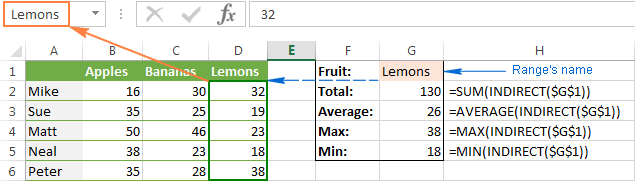Now that you've got the general idea of how to use the INDIRECT function in Excel, we can experiment with more powerful formulas.

### INDIRECT formula to dynamically refer to another worksheet

The usefulness of the Excel INDIRECT function is not limited to building "dynamic" cell references. You can also utilize it to refer to cells in other worksheets "on the fly", and here's how.

Suppose, you have some important data in Sheet 1, and you want to pull that data in Sheet 2. The following screenshot demonstrates how an Excel Indirect formula can handle this task: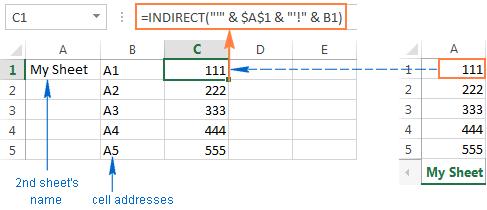Let's break apart the formula you see in the screenshot and understand.

As you know, the usual way to reference another sheet in Excel is writing the sheet's name followed by the exclamation mark and a cell / range reference, like SheetName!Range. Since a sheet name often contains a space(s), you'd better enclose it (the name, not a space : ) in single quotes to prevent an error, for example 'My Sheet!'\$A\$1.

And now, all you have to do is to enter the sheet name in one cell, the cell address in another, concatenate them in a text string, and feed that string to the INDIRECT function. Remember that in a text string, you have to enclose each element other than a cell address or number in double quotes and link all elements together using the concatenation operator (&).

Given the above, we get the following pattern:

INDIRECT("'" & Sheet's name & "'!" & Cell to pull data from)

Going back to our example, you put the sheet's name in cell A1, and type the cell addresses in column B, as demonstrated in the screenshot above. As the result, you get the following formula:

`INDIRECT("'" & \$A\$1 & "'!" & B1)`

Also, please pay attention that if you are copying the formula into multiple cells, you have to lock the reference to the sheet's name using the absolute cell references like \$A\$1.

Notes

• If either of the cells that contain the 2nd sheet's name and cell address (A1 and B1 in the above formula) is empty, your Indirect formula will return an error. To prevent this, you can wrap the INDIRECT function in the IF function:

`IF(OR(\$A\$1="",B1=""), "", INDIRECT("'" & \$A\$1 & "'!" & B1))`

• For the INDIRECT formula that refers to another sheet to work correctly, the referred sheet should be open, otherwise the formula will return a #REF error. To avoid the error, you can use the IFERROR function, which will display an empty string, whatever error occurs:

`IFERROR(INDIRECT("'" & \$A\$1 & "'!" &B1), "")`

### Creating an Excel dynamic reference to another workbook

The Indirect formula that refers to a different Excel workbook is based on the same approach as a reference to another spreadsheet. You just have to specify the workbook's name is addition to the sheet name and cell address.

To make things easier, let's start with making a reference to another book in the usual way (apostrophes are added in case your book and/or sheet names contain spaces):
'[Book_name.xlsx]Sheet_name'!Range

Assuming that the book name is in cell A2, the sheet name is in B2, and the cell address is in C2, we get the following formula:

`=INDIRECT("'[" & \$A\$2 & "]" & \$B\$2 & "'!" & C2)`

Since you don't want the cells containing the book's and sheet's names to change when copying the formula to other cells, you lock them by using absolute cell references, \$A\$2 and \$B\$2, respectively.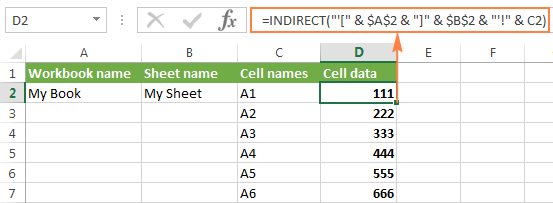And now, you can easily write your own dynamic reference to another Excel workbook by using the following pattern:

=INDIRECT("'[" & Book name & "]" & Sheet name & "'!" & Cell address)

Note. The workbook your formula refers to should always be open, otherwise the INDIRECT function will throw a #REF error. As usual, the IFERROR function can help you avoid it:

`=IFERROR(INDIRECT("'[" & A2 & "]" & \$A\$1 & "'!" & B1), "")`

### Using Excel INDIRECT function to lock a cell reference

Normally, Microsoft Excel changes cell references when you insert new or delete existing rows or columns in a sheet. To prevent this from happening, you can use the INDIRECT function to work with cell references that should remain intact in any case.

To illustrate the difference, please do the following:

1. Enter any value in any cell, say, number 20 in cell A1.
2. Refer to A1 from two other cells in different ways: `=A1` and `=INDIRECT("A1")`
3. Insert a new row above row 1.

See what happens? The cell with the equal to logical operator still returns 20, because its formula has been automatically changed to =A2. The cell with the INDIRECT formula now returns 0, because the formula was not changed when a new row was inserted and it still refers to cell A1, which is currently empty: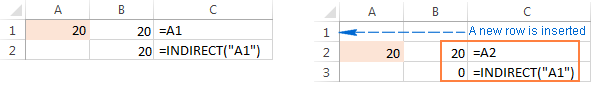After this demonstration, you may be under the impression that the INDIRECT function is more a nuisance than help. Okay, let's try it another way.

Suppose, you want to sum the values in cells A2:A5, and you can easily do this using the SUM function:

`=SUM(A2:A5)`

However, you want the formula to remain unchanged, no matter how many rows are deleted or inserted. The most obvious solution - the use of absolute references - won't help. To make sure, enter the formula `=SUM(\$A\$2:\$A\$5)` in some cell, insert a new row, say at row 3, and… find the formula converted to `=SUM(\$A\$2:\$A\$6)`.

Of course, such courtesy of Microsoft Excel will work fine in most cases. Nevertheless, there may be scenarios when you don't want the formula to get changed automatically. The solution is to use the INDIRECT function, like this:

`=SUM(INDIRECT("A2:A5"))`

Since Excel perceives "A1:A5" as a mere text string rather than a range reference, it won't make any changes when you insert or delete a row(s).

### Using INDIRECT with other Excel functions

Apart from SUM, INDIRECT is frequently used with other Excel functions such as ROW, COLUMN, ADDRESS, VLOOKUP, SUMIF, to name a few.

#### Example 1. INDIRECT and ROW functions

Quite often, the ROW function is used in Excel to return an array of values. For example, you can use the following array formula (remember it requires pressing Ctrl + Shift + Enter) to return the average of the 3 smallest numbers in the range A1:A10:

`=AVERAGE(SMALL(A1:A10,ROW(1:3)))`

However, if you insert a new row in your worksheet, anywhere between rows 1 and 3, the range in the ROW function will get changed to ROW(1:4) and the formula will return the average of the 4 smallest numbers instead of 3.

To prevent this from happening, nest INDIRECT in the ROW function and your array formula will always remain correct, no matter how many rows are inserted or deleted:

`=AVERAGE(SMALL(A1:A10,ROW(INDIRECT("1:3"))))`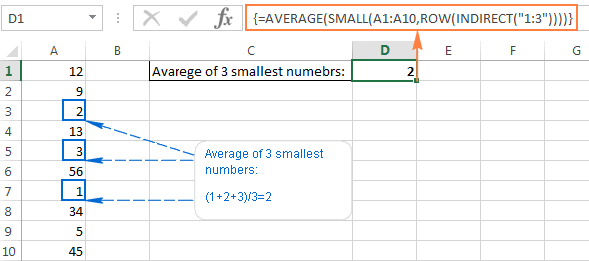Here's a couple more examples of using INDIRECT and ROW in conjunction with the LARGE function: How to sum N largest numbers in a range.

#### Example 2. INDIRECT and ADDRESS functions

You can use Excel INDIRECT together with the ADDRESS function to get a value in a certain cell on the fly.

As you may remember, the ADDRESS function is used in Excel to get a cell address by the row and column numbers. For example, the formula `=ADDRESS(1,3)` returns the string \$C\$1 since C1 is the cell at the intersection of the 1st row and 3rd column.

To create an indirect cell reference, you simply embed the ADDRESS function into an INDIRECT formula like this:

`=INDIRECT(ADDRESS(1,3))`

Of course, this trivial formula only demonstrates the technique. And here are a few examples that may prove really useful:

### Using INDIRECT with Data Validation in Excel

You can use the Excel INDIRECT function with Data Validation to create cascading drop down lists that display different choices depending on what value the user selected in the first dropdown.

A simple dependent drop-down list is really easy to make. All it takes is a few named ranges to store the dropdown's items and a simple `=INDIRECT(A2)` formula where A2 is the cell displaying your first drop-down list.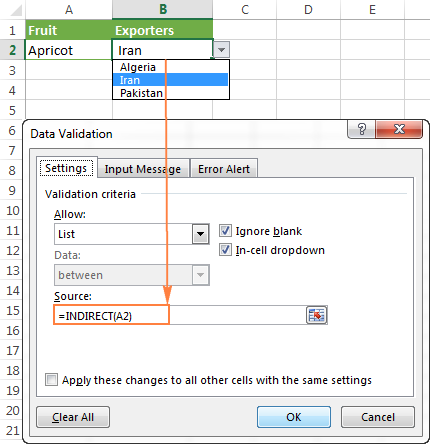To make more complex 3-level menus or drop-downs with multi-word entries, you will need a bit more complex INDIRECT formula with a nested SUBSTITUTE function.

For the detailed step-by-step guidance on how to use INDIRECT with Excel Data Validation, please check out this tutorial: How to make a dependent drop down list in Excel.

## Excel INDIRECT function - possible errors and issues

As demonstrated in the above examples, the INDIRECT function is quite helpful when dealing with cell and range references. However, not all Excel users eagerly embrace it mostly because extensive use of INDIRECT in Excel formulas results in lack of transparency. The INDIRECT function is difficult to review since the cell it refers to is not the ultimate location of the value used in the formula, which is pretty confusing indeed, especially when working with large complex formulas.

In addition to the above said, like any other Excel function, INDIRECT may throw an error if you misuse the function's arguments. Here's a list of most typical mistakes:

#### Excel INDIRECT #REF! error

Most often, the INDIRECT function returns a #REF! error in three cases:

1. ref_text is not a valid cell reference. If the ref_text parameter in your Indirect formula is not a valid cell reference, the formula will result in the #REF! error value. To avoid possible issues, please check the INDIRECT function's arguments.
2. Range limit is exceeded. If the ref_text argument of your Indirect formula refers to a range of cells beyond the row limit of 1,048,576 or the column limit of 16,384, you will also get the #REF error in Excel 2007, 2010 and Excel 2013. Earlier Excel versions ignore the exceeded limit and do return some value, though often not the one you'd expect.
3. The referred sheet or workbook is closed. If your Indirect formula refers to another Excel workbook or worksheet, that other workbook / spreadsheet must be open, otherwise INDIRECT returns the #REF! error.

#### Excel INDIRECT #NAME? error

This is the most obvious case, implying that there is some error in the function's name, which leads us to the next point : )

#### Using the INDIRECT function in non-English locales

You may be curious to know that the English name of the INDIRECT function has been translated into 14 languages, including:

 Danish - INDIREKTE Finnish - EPÄSUORA German - INDIREKT Hungarian - INDIREKT Italian - INDIRETTO Norwegian - INDIREKTE Polish - ADR.POŚR Spanish - INDIRECTO Swedish - INDIREKT Turkish - DOLAYLI

A common problem with non-English localizations is not the INDIRECT function's name, but rather different Regional Settings for the List Separator. In the standard Windows configuration for North America and some other countries, the default List Separator is a comma. While in European countries, the comma is reserved as the Decimal Symbol and the List Separator is set to semicolon.

As a result, when copying a formula between two different Excel locales, you may get the error message "We found a problem with this formula…" because the List separator used in the formula is different from what is set on your machine. If you run into this error when copying some INDIRECT formula from this tutorial into your Excel, simply replace all commas (,) with semicolons (;) to get it fixed.

To check which List Separator and Decimal Symbol are set on your machine, open the Control Panel, and go to Region and Language > Additional Settings.

Hopefully, this tutorial has shed some light on using INDIRECT in Excel. Now that you know its strengths and limitations, it's time to give it a shot and see how the INDIRECT function can simplify your Excel tasks. Thank you for reading!

## You may also be interested in

1. In one workbook I have two worksheets: Analysis and Items.Items contains detail line items sold with a customer ID in column N. There may be one or many rows with the same customer ID. In Analysis WS I want a count of when the customer ID changes in Items WS(counting number of customers).Using Countifs function as there are multiple criteria. Want to compare value of N to N of previous row. Can you help? Tried Index and Offset, but no luck.

2. Hi ,
SOS. I have non contiguous cells on one sheet ( different sheets) and i want to sum or average them using indirect function. How does it work ? Many thanks.

As you might have noticed in the examples, AVERAGE and SUM functions are used with INDIRECT when it is referred to a cell with already named range. So you will most likely have to create a long INDIRECT formula, creating additional cell references for your non contiguous cells from different sheets.
You can find it explained in more details here.

3. I'm having trouble getting "Creating an Excel dynamic reference to another workbook" to work. I type this in verbatim - nothing but REF#.

I understand the big picture regarding how it works. I also have the source sheet open. I tried closing and re-opening the source workbook again, no good.

Any assistance would be deeply welcomed and appreciated.

• Nevermind, I figured it out. I needed to type the following: =INDIRECT("'["&\$A\$2&".xlsx]"&\$B\$2&"'!"&C2)

4. I am using the INDIRECT function to pull data from another tab in the current worksheet. When I edit the location I get the REF error. When I cut and paste the same text it works?

5. =COUNTIF(D6:D31,1,("1=Trivandrum"),("2=Kollam"),("3=Pathanamthitta")

6. =COUNTIF(D6:D31,1,("1=Trivandrum"),("2=Kollam"),("3=Pathanamthitta")

7. Hi Svetlana Cheusheva

A1 jhon
A2 krish
A3 robert

B1 50
B2 100
B3 150

now i need formula for

Column D jhon 50
Column E krish 100
Column F robert 150
Auto insert value of persons

8. you can comple this please, and explain my more , indirect(adresse(count())) help do it.

9. Hello,
Not sure if something changed from the time this was posted but I found a bug in your syntax for the sheet reference example on my system.

You listed the following syntax INDIRECT("'" & \$A\$1 & "'!" & B1)
But that yields #REF error until the last quote is moved right of the cell address "B1". At least from my test on Office2016 your example fails until I do this...

INDIRECT("'" & \$A\$1 & "'!" & B1) BROKEN
INDIRECT("'" & \$A\$1 & "'!B1") WORKING

10. Hi Svetlana,

I was so intrigue by your article, would it be possible to send me an example sheet so that I could download it and use it in my work.

Thank so much, Edward

How to use INDIRECT function in Excel - formula examples

1,2,3,4,5,6,7,8,9,10,...etc have magic number and models in customer list and I want to reference like that

12. =IFERROR(HLOOKUP(\$B\$7,INDIRECT("'["&\$C\$1&"]"&\$A\$3&"'!"&\$C\$18:\$CV\$55),38,FALSE),"")

I'm trying to pull data from another workbook on our SharePoint site.

C1 = "http://blueshare/Departments/Finance/Budget" and Rates/Billing/Generation Partners Workpapers/2016/09 - Generation Partners Workbooks September 2016/Residential Worksheet September 2016.xlsx
A3 = Data Input Worksheet

I'm typing in the range: C18:CV55.

Please tell me why it's not working. Thanks.

• It looks like you are putting the entire path in square brackets. Shouldn't just the file be in square brackets?

And because there are spaces in the filepath you might need to add single quotes around the whole file name. Have a look at the Creating an Excel dynamic reference to another workbook for the correct syntax.

13. Good day and thank you for helping all of us!
I have an issue and I'd be so thankful if you could help me.
I have a spreadsheet (daily data) that pulls data from a closed workbook.
I have a folder (auto) with data from every day as a different workbook. Nov 09.xlsx, Nov 10.xlsx, Nov 11.xlsx, etc.

in my example, the data is shown in cell V21 in my 'daily data' spreadsheet. the formula for V21 is ='I:\DOD\Intervals\auto\[Nov 09.xlsx]Daily DOD'!\$F21

Now, what I need is the ability to use another cell in my 'daily data' spreadsheet, where I type the date I need the formula in V21 to pull data from.

so I want to use cell A1 to type a date (Nov 09 in this case) and have cell V21 go to 'I:\DOD\Intervals\auto\[Nov 09.xlsx]Daily DOD'!\$F21 and give me that data. but if I change the date in A1 to, say Dec 10, I want cell V21 to give me the data in 'I:\DOD\Intervals\auto\[Dec 10.xlsx]Daily DOD'!\$F21 instead.
Is there a way to concatenate the date in A1 to the formula in V21? or is there other function/ formula I could use to automate this process, instead if changing the formula in V21 manually every time I change the date I want to see?

Thanks so much!

14. I need help with an indirect formula. I have drop-down two columns, say A and B. If the choice "Pres" "Corres" or "Other" is selected in column A, I want column B to display the relevant drop-down listing.

My indirect formula works exceptional. However, the problem I'm having is that when I enter "Other" I want the cell in Column B to able to input my own information. And because I don't have any info in the drop-down for "Other" it's coming up blank.

Is there a way for the dropdown to occur for only "Pres" and "Corres" only and allow text for "Other"?

15. Hi Svetlana Cheusheva

I need help in the following data. on the basis of multiple conditions I want to put a Text value (Result). Like as per Voucher Dt., CHQ Dt., CF Report and Status the text value should come accordingly.

suppose if voucher dt. (Specific month dt) + chq dt.(Specific month dt) + CF Report (Specific condition) + Status (Specific Status Name) then Result (Text Name) should come on the basis of all specific conditions.

Voucher Dt. CHQ. Dt. Name Amt CF Report Status Result
15-08-16 15-08-16 ABC 500.00 Chq in Hand Received CDC
20-06-16 05-08-16 DEF 400.00 Chq in Hand Not Received PDC
21-06-16 05-08-16 GHI 500.00 Factoring Received DISCOUNT CHQ
25-08-16 25-08-16 JKL 300.00 Cash in Hand Received CASH
06-08-16 06-08-16 MNO 400.00 Chq in Hand Not Received CDC

16. Hi,
I'm trying to combine INDIRECT with a 3D consolidation, doesn't seem to be working:
Sheet: A, B and C
each have :
A1 = 1 for sheet A
A1 = 2 for sheet B
A1 = 3 for sheet C
if I do:
=sum(A:C!A1) then I get: 6

but if I do: =sum(indirect(A:C!A1))
then I get an error...

Any idea ?

17. explain the formula =iferror(index(dddd,small(if(dddd"",row(indirect("1:"&rows(dddd)))),row(indirect("1:"&rows(dddd)))),"")

18. I'm using the following formula and when i insert a row in the source worksheet, the cell reference should change to F57 but it does not.

(INDIRECT("'"&A2&"'"&"!" &"F56")

• It doesn't change because "F56" is interpreted as text when placed between the quotes"" not as a cell reference. Thus changes to rows columns do not impact it.

You will see the A2 shift because it is placed as a cell ref between the &&. I am battling a similar problem where I want the "F56" to increment but it wont. In the example in the article the author lists the following syntax (INDIRECT("'"&A2&"'"&"!"&F56). This would allow F56 to increment but it is fundamentally incorrect because it is trying to place the contents of F56 on the current sheet into the string rather than appending just the letter F and number 56 to the string for later lookup in the proper sheet. Let me know if you find the answer.

• Any fix on this one yet?

19. after applying indirect function on data if some data is added is this function applied to that data..i checed that but it is not taking the newly added data ..
thank u

20. Ablebits.com i love you too much in-fact you've made me like and understand excel so much thank u.

21. Excellent article...

Dik

22. I have two sheets Sheet1 and Milk, I intend to get data from sheet "Milk" sheet1. I "=Milk!\$AJ30" put this in formula bar, i need to get fixed column but varied row in Sheet1. I could not get the desired result.
I only get fixed column AJ and same Row 30 every time when I drag by the handle. it works vertically not horizontally.
i want result in the rows.
Regards
Qamar

23. When I try =SUM(INDIRECT(\$A22&"!\$C\$3")) which has text in (\$C\$3) it comes back with 0.
Any ideas on how to get the text from that sheet to display. :)

Robert this error may be because you are NOT using sheet reference.

Let says your sheet name is "XYZ". IN any sheet put A1- XYZ

Then use indirect like INDIRECT(A1&"!"&"a2:b3")....
Here A1 will refer to XYZ
! refer to XYZ!
A2: B3 : if lets say is your sheet range.

This will give you sheet reference

24. Wow thank you.. You re genius.. Really help

25. sheet name is x,
I have a column name Ex: 7(g:g),

I want to use Indirect with sheetname (X) and column number 7(g:g), column number will be changed 8 and 9 and 10.... any idea...

26. Hello,
my question is the following :

I wish to use the cells in column E to reference the value of its correlative C cell WHEN the value in the E cell is 1.

In the F1 cell, is where I would like for this value to appear.

I have tried with the INDIRECT function, and I think it's the most probable answer, but I can't seem to get it quite right.
e.g.

In F1 must appear value in C2 if E2=1 (if E2 is blank or 0, omit)
In F1 must appear value in C3 if E3=1 (if E3 is blank or 0, omit)
In F1 must appear value in C4 if E4=1 (if E4 is blank or 0, omit)
and so on...

Is there any simple formula to do this?

27. I am trying to use Indirect to get text from a cell in another sheet
=SUM(INDIRECT(\$A22&"!\$B\$3")) gets me the date (\$B\$3) that is in the sheet name in (\$A22).
When I try =SUM(INDIRECT(\$A22&"!\$C\$3")) which has text in (\$C\$3) it comes back with 0.
Any ideas on how to get the text from that sheet to display. :)

• What do you expect the "sum" of "text" to be? I am not sure if I am more concerned that you are trying to sum a single cell or that you are trying to sum text. What is the cube root of banana?

If you remove the sum function it will return the text.

28. Using indirect address with match sum up the salary for respective months.start month is Feb and end month is june
A salary
Jan4000
Feb2000 stare end totsal
Mar1000 feb june
Apr5000
May3000
June 1ooo

29. Buenas noches

estoy tratando de aplicar la formula =INDIRECT(SUBSTITUTE,(B2,"",")) pero al momento de darle aplicar el sistema no me la reconoce me podrian colaborar por favor gracias

30. There are no quirk in Excel with indirect function.
You misunderstood the indirect(R3C) reference. As the function is written in Cell D5 (row3, colonne4) it directly go and catch the value in (Row3, colonne4)

indirect(R3C4) = is looking for Row3 Colonne4
When no reference to the number of the column is done (indirect(R3C) then it refers to the column where the formula (look for which cell the equal(=) sign is)

Also you should change your blue array in you sreenshot. The array from C5 to C3 should be change into C5 to D3

As a conclusion the formula in D5: "=Indirect(C5,false)" return the value of R3C3 as expected (ie "R2C1")

If you want the indirect to go further and catch 222 (Cell A2) then you should have written:
=INDIRECT(INDIRECT(C5,false),false)

31. by using Concatenate function, how we further write in the resulted cell because resulted cell shows the formula nor text.

• Hello, Ali,

32. Just perfect! Thanks!

33. Hi Svetlana,

Is that possible to use indirect function to dynamically change the row number i.e. =INDIRECT(\$A\$4&"!C31"), =INDIRECT(\$A\$4&"!C32") and so on.

• Hi Taha,

You can use the formula below:
=INDIRECT(A4 &":C31")

• Hello Taha,

I get #ref! error when doing this. If I take the : out then it returns a value but does not increment when I try to cascade the formula. But even with the : in place it does not increment like the original question asked about.

34. I have a small problem
in cell A1 - birthdate 01/01/2010
in cell B1 - =A1 : 01/01/2010
when I try with indirect I get the number instead of a date format

My goal is put in one cell indirect bithdate + birthplace
A1 & " " & A2

I hope you have a great holiday, and looking forward to your fresh look at this issue

• Hi, Kris,

Try this:

text(A1,"DD/MM/YYYY")&" "&A2

If you want the date in American format, use "MM/DD/YYYY".

35. Employee sheet details
Employee ID Group Name Machine Name Uniq ID Start Date End Date NumberOfAssets Machine Added to Domain
8002 IT Navaneeth.Sarvamanya nsarvamanya 8002-nsarvamanya - - 2 Y
8016 Engineer Suraj Bhasin sbhasin 8016-sbhasin - - 3 Y

Inventory sheet details
Type Model # Serial # Uniq ID Procurment Date End Date Hardware Condition Status
Laptop Official Z930 1E137603H Z930-1E137603H - - Available
Laptop Official Z930 3E099576H Z930-3E099576H 01-06-2014 31-05-2017 Lost

AssetLog sheet details

Start Date End Date Asset Employee Name Remarks Hardware Condition
01-02-2013 - Z930-1D094026H 8001-achhabra Working

Issue in the formulae
ActiveCell.Value = "=INDEX(Inventory!\$E\$6:\$E\$264,MATCH(""Management""&""Z930*""&""Available"",INDIRECT(""Employee!""&(""c""&ro))&Inventory!\$E\$6:\$E\$264&Inventory!\$I\$6:\$I\$264,0))"

ro is the variable which is row number which is specified; ro is not getting resolved hence I am getting #Name can you suggest me how to solve this issue?

36. Help! What is wrong with this formula?

=(COUNTIFS('Prod - Resolved'!(INDIRECT("B3:B500")),"1 - Critical"))

I'm trying to count the number of cells on the worksheet named Prod - Resolved, in the range B3 to B500, where the value is "1 - Critical".

Thanks!

• Hello, Kedra,

=(COUNTIF(INDIRECT("'Prod - Resolved'!B3:B500");"1 - Critical"))

37. Hi all,

I need your support. I have 3 drop down list where I have used indirect functions where box B depends on box A & box C depends on Box B and this have to work from A to C instead. I was able to lock A if is there any information on B ( I mean, box A not allow to filter only when box B is empty)
My concern is that I'm not able to lock box B for selection if the box C has information.

Regards

38. Hi I am creating a report spread sheet with a YES or NO box. If the box has YES in it I want he workbook to open another sheet or if NO then stay on the same sheet, is this possible.
Cheers Steve

39. "For example, you can use the following array formula (remember it requires pressing Ctrl + Alt + Enter)"

Ctrl+Alt+Enter ???? I don't think so.

• Hi Sandy,

Of course, it's CTRL+SHIFT+ENTER. Don't know how Alt could sneak in. Thanks for catching it!

40. i want to indirect to another sheet but show the rows that have specific criteria

41. I am working on a calculation sheet where i have three different set of inputs and values for the three types of sub categories for example: I have a fruits business where i sell apple, orange, banana. All the three fruits have a different sales and purchase prices and so on. I have created a drop down list for the tree fruits and selected a fruit. As i have selected one fruit i wanted to display the respective calculations in the below cells. If I again change the input the values in the drop down the displayed values below should change accordingly. Can this be possible?

42. hi,
how can i get the value of specific cell value of workbook that when it closed with indirect(without VBA)?

43. Thank you for the above information. It looks like I am doing this wrong by not using named ranges but I would like to ask anyways.

I'm working on a project that requires a separate "data" workbook and a number of "template" workbooks that are applied to the data workbook to create new user workbooks on the fly. My question is about using indirect to point to tables.

In the template workbooks I have a number of formulas that are pointing to the data workbook. When I create a merged user workbook by adding the template workbook to the data workbook, I'm am using VBA to edit out the file pointers to the data workbook so that the new workbook will not be reaching out to the original data workbook, becoming self contained.

Specifically the template workbook has the data validation list that uses Indirect to successfully to point to the data workbook:
=INDIRECT("Data.xltm!tblContacts[Name]")

After the worksheet is copied to the data workbook the edited Formula1 value is:
=INDIRECT("tblContacts[ContactName]")

However I'm getting a 1004 error on this code when I try to update the cell:
Cell.Activate
With Cell.Validation
.Delete
End With

Any ideas?

Thank you

44. i have a doubt in ms excel. In a column there are many cells in that column i want to take uncoloured cells from that column trough any equation. would you please help me to do it.

45. I have a drop down box at the top of "summary" worksheet that has the names of all the other worksheets in the workbook. In a later cell, I am trying to use INDIRECT to reference a cell on the sheet that comes from the drop down box, but I can't figure it out. (all the other sheets are formatted the same, so the data I'm looking for is always in the same cell) This way when I select what worksheet I want (from the drop down box), the data automatically fills in down the summary sheet.
Latest attempt: =INDIRECT("'"&A1&"'!"D10)

46. I am trying to use indirect to execute a DDE command based on variable. the original command is ='MT4'|HIGH!EURUSD

I want to create that command in a a cell then execute it in another cell.

47. Hello Svetlana,

many thanks for refering to my Excel-Translator.de in your post :-)

Best Regards

48. I was badly looking for that function
Really thx

49. Hello Miss, i am trying to use indirect function for the file that is closed but it dose not work unless file is open can you help. filename Account.xlsx DATA:
A5 = F:\DATA\INVOICES, A6 = 1385_02/14/2015, A7 = Basicinvoice, A8 = \$i\$37
FORMULA: C1 = =INDIRECT("'"&A5&"["&A6&".xlsx]"&A7&"'!"&A8)

• Hi Aziz,

I am afraid this cannot be fixed. When an Indirect formula refers to another Excel file, it must be open, otherwise you will get the #REF! error.

• How to do the formuala to use in another workbook indirect function when will drag down , sheet number changed and the data changed.

50. I have your product and love it. Use Merge Tables but have not had cause to explore other tools. Now I have issue where formulas turn to reference if rows are deleted after Table Merge in tab that is reference. Tried absolute and not success. Tried using Indirect Function which work for deletion of reference ROW issue but it doesn't let me drag formulas down page to populate my data for export. Was trying CHAR AND ROW FUNCTIONS but unable to make ASCII character for AA, which would be 65,65. It seems like I'm missing a simple answer. Any help would be appreciated.

• Hello Mike,

I am sorry it's hard to determine the cause of the problem without seeing your data. If you could post this question on our forum and attach a sample forkbook, our support team will try to help.